# Use LM393 IR Module as Motor Speed Sensor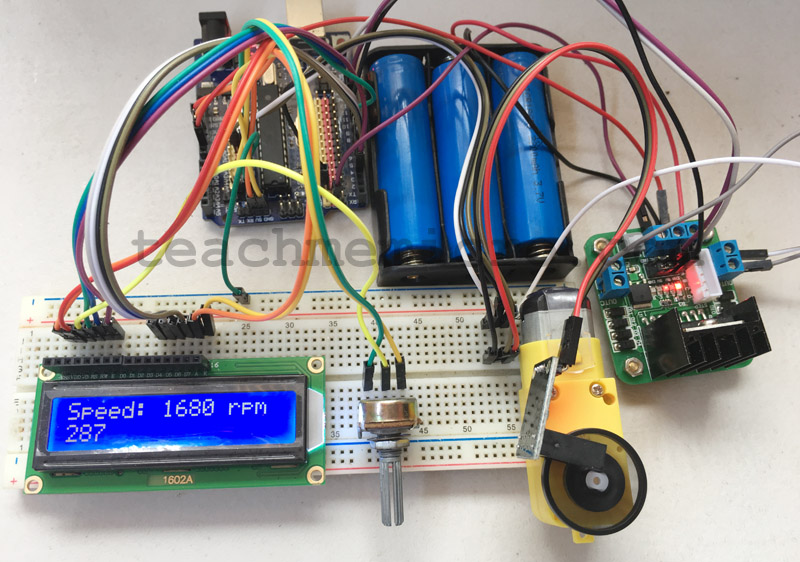Unlike stepper and servo motors, precision control for DC motors is impossible unless a sort of feedback mechanism is employed. If your application requires a consistent motor speed or a stimulant-dependent speed then you certainly need feedback. This tutorial aims to develop a simple motor speed sensor for a more intelligent DC motor control.

### The LM393 IR Module

For this tutorial, I will use this inexpensive module: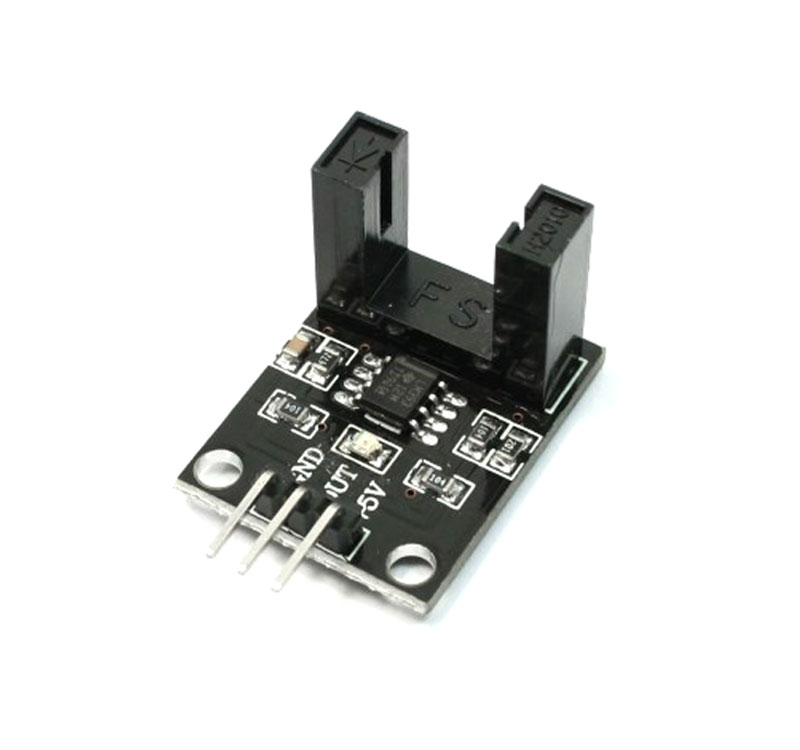The module has two vertical columns with an IR LED on one column and a phototransistor on the other. Whenever the path between the IR LED and phototransistor is cut, the D0 pin goes high.

Sellers call this module a LM393 speed sensor. The LM393 is in fact a comparator IC and not a sensor, per se. The module schematic is as follows: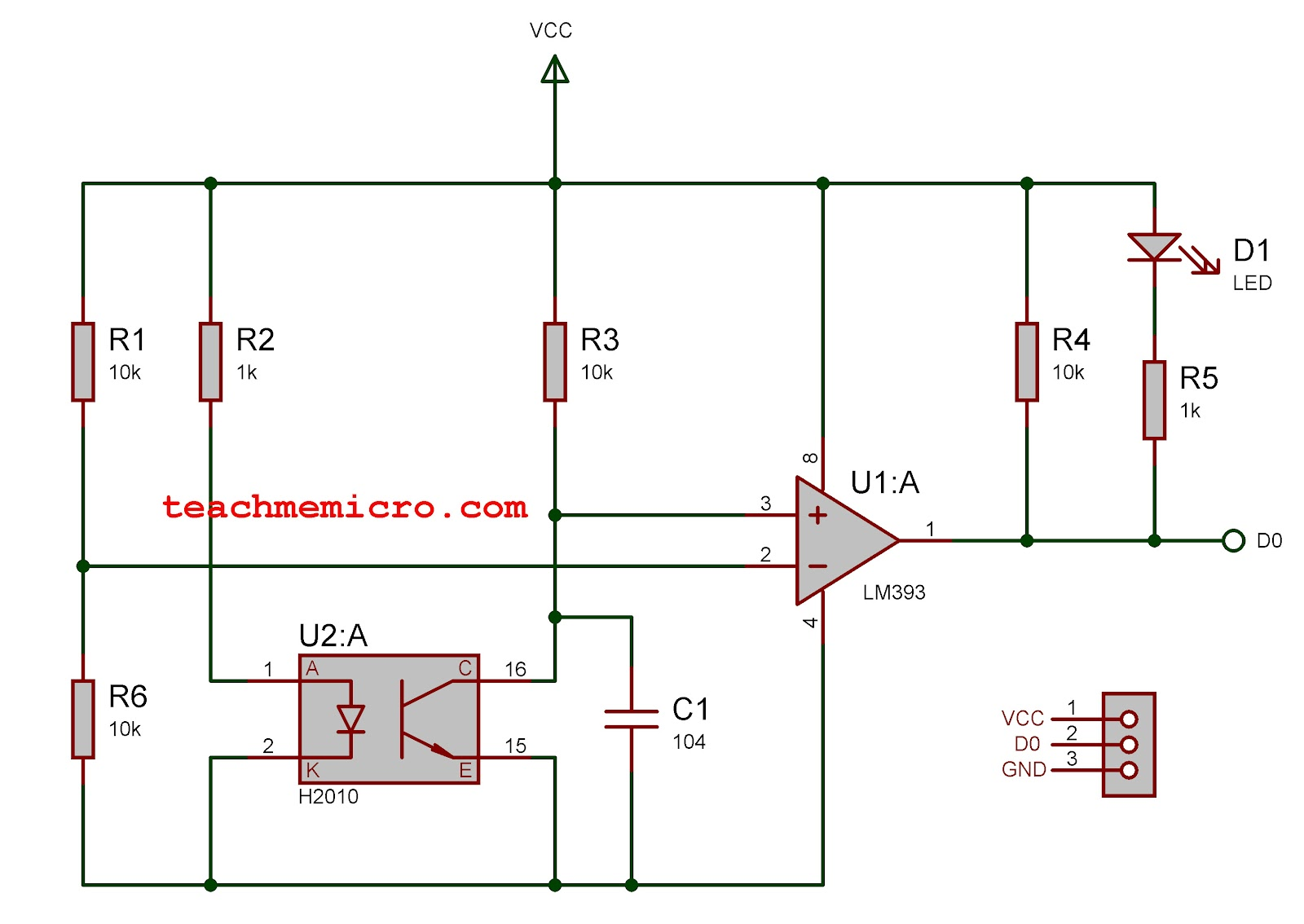Here we see how simple the circuit for the module is. With no obstruction between the IR LED and phototransistor, the voltage between the positive and negative terminals of the comparator is equal. When the phototransistor is blocked, it will draw a higher voltage, making the positive terminal of the comparator more positive than the negative terminal. Thus, a positive voltage, equal to VCC, will be at the D0 terminal.

### Determining Motor Speed

A wheel encoder on the DC motor will help us determine its speed: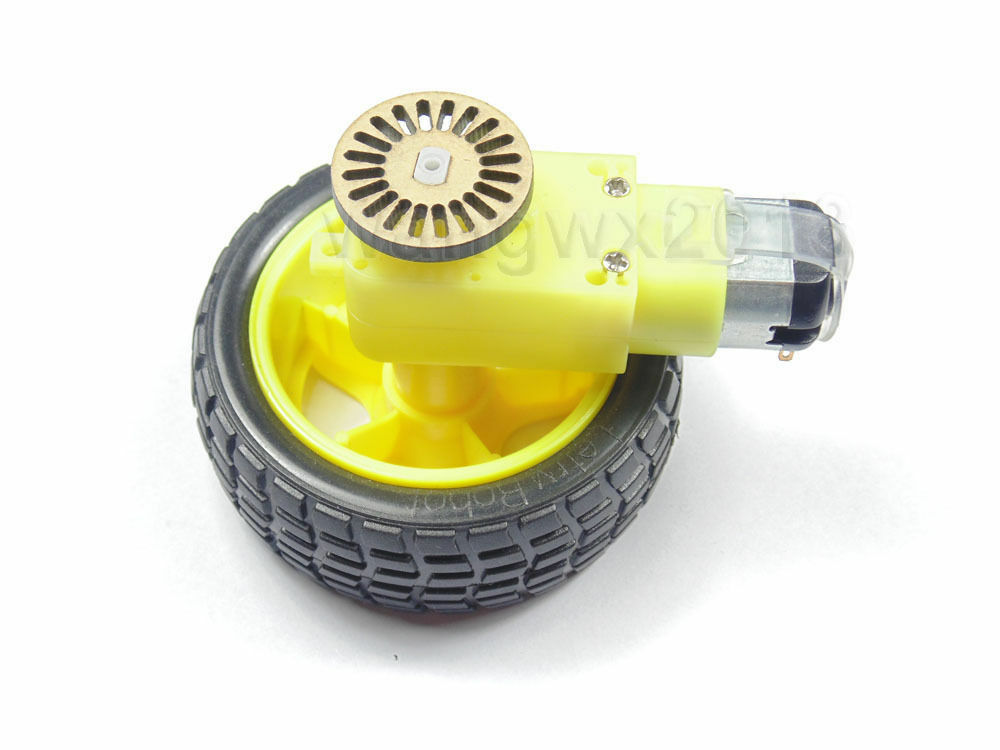With proper placement, the slots on the encoder will block or pass the IR LED. This will create a train of pulses whose frequency is proportional to the speed of the motor.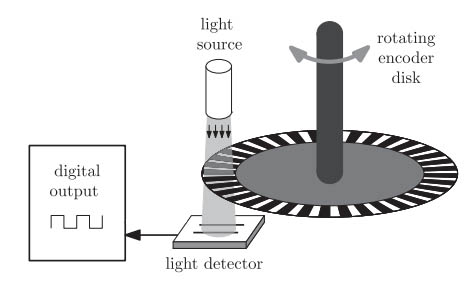The wheel encoder pictured has 20 slots. Therefore, counting 20 pulses mean the wheel has traveled one revolution.

If we attach the D0 pin of the module to interrupt 0 of the Arduino, which is digital pin 2, we can count the number of pulses if we have this as the interrupt service routine:

```attachInterrupt(0, pulsecount, RISING);

void pulsecount(){
counter++;
}```

The number of revolutions will then be:

Motor speed is normally in revolutions per minute or RPM. Since we have an idea on determining how many revolutions the motor has travelled, all we need is the check the number of revolutions every minute. We can do that through the help of the millis() function inside the loop():

```int rpm;
void loop(){
static uint32_t previousMillis;
if (millis() - previousMillis >= 1000) {
rpm = (counter/20)*60;
counter = 0;
previousMillis += 1000;
}
}```

Here, we calculate the RPM every 1 second and then reset the pulse counter to 0.

### Motor Speed Sensor Project

Using a L298N motor driver, we follow this connection for testing our motor speed sensor:

Arduino LCD L298N Module LM393 Module
8 RS
3 EN
GND RW
7 D4
6 D5
5 D6
4 D7
12 IN1
13 IN2
9 ENA
2 D0
5V 5V VCC
GND GND GND

I used three 18650 LiPo batteries as power source. The L298N module has a 5V regulator so this is where the Arduino, LCD and LM393 module get their power. The DC motor takes on the 11.1 volts from the LiPo batteries.

The speed in RPM is viewable on a 16 x 2 LCD. Here is the full sketch:

```#include <LiquidCrystal.h>

int motorIn1 = 12;
int motorIn2 = 13;
int motorEnA = 9;

int encoder = 2;

volatile unsigned int counter;
int rpm;

const int rs = 8, en = 3, d4 = 7, d5 = 6, d6 = 5, d7 = 4;

LiquidCrystal lcd(rs, en, d4, d5, d6, d7);

void setup() {
pinMode(motorIn1, OUTPUT);
pinMode(motorIn2, OUTPUT);
pinMode(motorEnA, OUTPUT);
pinMode(encoder, INPUT);

digitalWrite(encoder, HIGH);
digitalWrite(motorIn1, HIGH);
digitalWrite(motorIn2, LOW);
analogWrite(motorEnA, 100);

attachInterrupt(0,countpulse,RISING);
lcd.begin(16, 2);
}

void countpulse(){
counter++;
}

void loop() {
static uint32_t previousMillis;
if (millis() - previousMillis >= 1000) {
rpm = (counter/20)*60;
counter = 0;
previousMillis += 1000;
}

lcd.setCursor(0,0);
lcd.print("Speed: ");
lcd.setCursor(7,0);
lcd.print(rpm);
lcd.print(" rps");
delay(1);
}```

This video shows the result of the sketch:

By knowing the speed of the motor, we can now use it as feedback for a PID controller to maintain its speed.